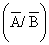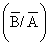Tuesday 29th September 2020CBSE Guess > Papers > Important Questions > Class XII > 2009 > Maths > Mathematics By Anil Kumar Tondak

CBSE CLASS XII

Probability

Q. 1. A die is thrown twice and the sum of the numbers appearing is observed to be 6. What is the conditional probability that the number 4 has appeared at least once.

Q. 2. Assume that each born child is equally likely to be a boy or a girl. If a family has two children, what is the conditional probability that both are girls given that at least one is a girl.

Q. 3. If A and B are two independent events, show that the probability of occurrence of at least one of A and B is given by : 1 – P(A').P(B')

Q. 4. Probability of solving specific problem independently by A and B are ½ and 1/3 respectively. If both try to solve the problem independently, find the probability that the problem is solved.

Q. 5. An urn contains 5 red and 5 black balls. A ball is drawn at random, its colour is noted and is returned to the urn. Moreover, 2 additional balls of the colour drawn are put in the urn and then a ball is drawn at random. What is the probability that the second ball is red.

Q. 6. Two cards are drawn simultaneously (or successively without replacement) from a well shuffled pack of 52 cards. Find the  mean, variance and standard deviation of the number of kings.

Q. 7. In an examination, 20 questions of true-false type are asked. Suppose a student tosses a fair coin to determine his answer to each question. If the coin falls heads, he answers ‘true’, if it falls tails, he answers ‘false’. Find the probability that he answers at least 12 questions correctly.

Q. 8. A die is thrown again and again until three sixes are obtained. Find the probability of obtaining the third six in the sixth throw of the die.
Q. 9. If P(A) = 3/8,  P(B) = ½ and P (AÇB) = ¼,     find Pand  P.

Q. 10. The probability that a certain person will buy a shirt is 0.2, the probability that he will buy a trouser is 0.3 and the probability that he will buy a shirt given that he buys a trouser is 0.4. Find the probability that he will buy both a shirt and a trouser. Find also the probability that he will buy a trouser given that he buys a shirt.

Q. 11. A can solve 90% of the problem given in a book and B can solve 70%. What is the probability that at least one of them will solve the problem, selected at random from the book.

Q. 12. Three persons A, B, C throw a die in succession till one gets a ‘six’ and wins the game. Find their respective probabilities of winning, if A begins.

Q. 13. A man is known to speak truth 3 out of 4 times. He throws a die and reports that it is a six. Find the probability that it is actually a six.

Q. 14. An urn contains 4 white and 3 red balls. Find the probability distribution of the number of red balls in a random draw of three balls.

Q. 15. In a meeting 70% of the members favour a certain proposal,30% being opposed. A member is selected at random and let X=0 if he opposed and X =1 if he is in favour. Find E(x) and Var(x).

Q. 16. Find the probability distribution of the number of doublets in 4 throws of a pair of dice.

Prepared By Anil Kumar Tondak
M.Sc.(Maths), M.Ed., M.A.(Eco),M.B.A.(Mkt)
Mob No. 9811363962

 Maths